# 傅里叶变换与快速傅里叶变换

1、首先离散傅里叶变换目的 简单点说： 就是将一个信号从时域变换到频域

标准点说： 将以时间为自变量的信号 与 频率为自变量的频谱函数之间的某种关系变换$\hat{x}[k]=\sum_{n=0}^{N-1} e^{-i\frac{2\pi}{N}nk}x[n] \qquad k = 0,1,\ldots,N-1.$$\hat{x}=\mathcal{F}x$$x\left[n\right]={1 \over N}\sum_{k=0}^{N-1} e^{ i\frac{2\pi}{N}nk}\hat{x}[k] \qquad n = 0,1,\ldots,N-1.$$x=\mathcal{F}^{-1}\hat{x}$

S=2+3*cos(2*pi*50*t-pi*30/180)+1.5*cos(2*pi*75*t+pi*90/180)

现在对该信号做N = 512 点快速傅里叶变换：观察其幅度频率特性 和相位频率特性；

要说明的是采样点数会影响频率分辨率的 例如 抽样频率为Fs  = 1024 Hz 采样点数N = 1024 那么频率分辨率为 1Hz  如果N = 512 那么采样频率为 2Hz，假设原信号的幅值为 Ac  并且经过FFT 变换后的结果每个点的幅值 是Ac*(N/2) (除了直流分量) 模值是直流分量的 N 倍

close all;
A1 = 3; %频率 F1信号的幅度
A2 = 1.5; %频率为F2信号的频率
F1 = 50;
F2 = 75;
Fs = 256; %抽样频率为1024Hz
P1 = -30; %相位为 -30度
P2 = 90 ;%相位为90度
N= 256; %采样点数为1024
t = [0:1/Fs:N/Fs];

%信号
S = Adc + A1*cos(2*pi*F1*t + pi*P1/180) + A2*cos(2*pi*F2*t +pi*P2/180);
plot(S);   %显示原始信号
title('原始信号');

%对其做fft变换
figure;
Y = fft(S,N);
Ayy = abs(Y);
plot(Ayy(1:N)); %原始信号fft模值

%下面对其才进行幅频分析
figure;
Ayy = Ayy/(N/2); % 还原真实幅度值
Ayy(1) = Ayy(1)/2;
F =([1:N])*Fs/N;
plot(F,Ayy);
title('幅频特性');

%下面是相频特性
figure;
Pyy = [1:N/2] ; %为什么这里是1：N/2 因为每次采样就是有一半是没有用的
for i= 1:N/2
Pyy(i) = phase(Y(i));
Pyy(i) = Pyy(i)*180/pi;  %并将器转换为角度
end
plot(F(1:N/2),Pyy);
title('相位频率特性曲线');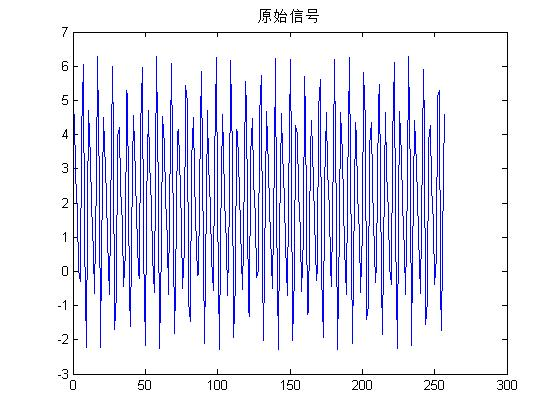2.fft变换后的赋值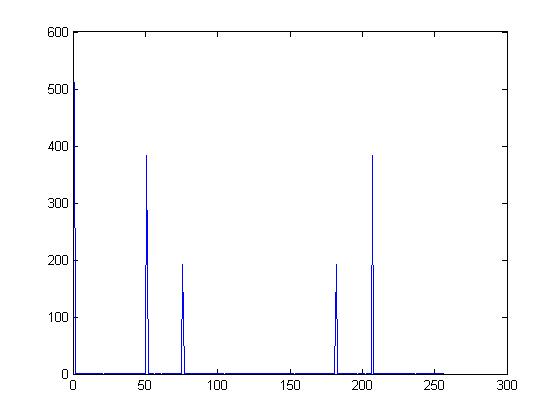3、信号的幅频特性：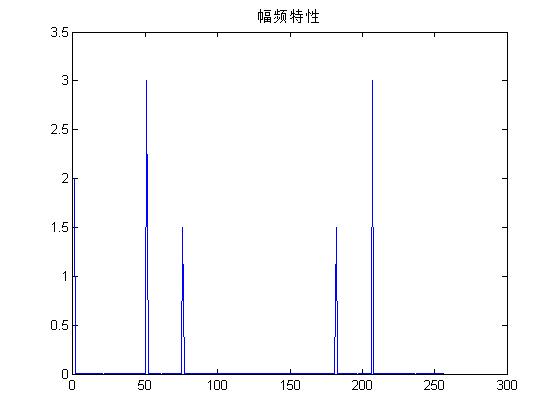4、相频特性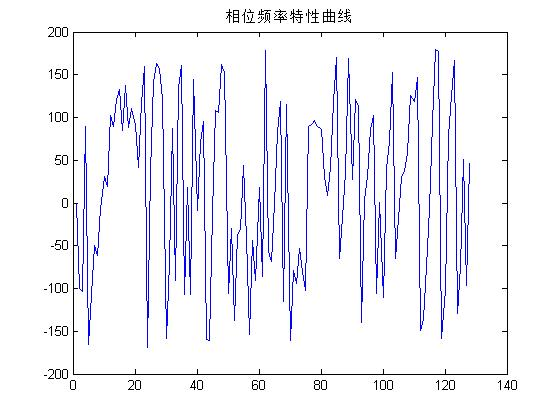wangxiaoming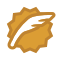CSDN认证博客专家 架构 Spring Boot Redis08-308万+

#### Matlab中fft函数的用法及关键问题详解

05-133万+

#### FFT在matlab中的使用方法

08-192741

#### MATLAB中FFT使用方法

02-221571

#### 使用 FFT 进行频谱分析©️2020 CSDN 皮肤主题: Age of Ai 设计师: meimeiellie点击重新获取扫码支付1.余额是钱包充值的虚拟货币，按照1:1的比例进行支付金额的抵扣。
2.余额无法直接购买下载，可以购买VIP、C币套餐、付费专栏及课程。余额充值